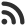Feed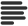Articles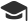Tutorials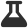Lab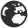CompaniesLeaderboard
 DG Student at BIT Mesra Aug. 24, 2020, 1:47 p.m. ⋅ 1051 views

# LTI placement technical questions with answer

1. Stack is useful for implementing:

A. Recursion
B. Depth first search
C. Both (A) & (B)

Ans. C

2. The least frequently used (LFU) page replacement algorithm requires_________

A. the page with the smallest count to be replaced.
B. the page with the longest count to be replaced.
C. the page with the smallest count was brought in, yet to be used.
D. the page with the longest count was brought in, yet to be used.

Ans. A

3. The time complexity of linear search algorithm over an array of n elements is

A. O (log2 n)
B. O (n)
C. O (n log2 n )
D. O (n2)

Ans. B

4. Which of the below functions is Not declared in math.h?

A. pow()
B. hex()
C. sqrt()
D. cos()

Ans. B

5. If malloc() fails to allocate the requested memory, it returns

A. Null
B. Garbage Value
C. Zero
D. None of the Mentioned

Ans. A

6. The time required to insert an element in a stack with linked list
implementation is

A. O (1)
B. O (log2 n)
C. O (n)
D. O (n log2 n )

Ans. A

7. The best data structure to check whether an arithmetic expression has balanced parenthesis is a

A. Queue
B. Stack
C. Tree

Ans. B

8. How to dynamically release memory?

A. Free()
B. Truncate()
C. delete()
D. release()

Ans. A

9. Reference is __________

A. alias of an object
B. used to rename an object
C. a special operator
D. a pointer to virtual function

10. A data type is stored as an 6 bit signed integer. Which of the following cannot be represented by this data type?

A. -12
B. 0
C. 32
D. 18

Ans. C

11. Stuti is making a questionnaire of True-false questions. She wants to define a data-type which stores the response of the candidate for the question. What is the most-suited data type for this purpose?

A. integer
B. boolean
C. float
D. character

Ans. B

12. Afzal writes a piece of code, where a set of three lines occur around 10 times in different parts of the program. What programming concept can he use to shorten his program code length?

A. Use for loops
B. Use functions
C. Use arrays
D. Use classes

Ans. B

13. A language has 28 different letters in total. Each word in the language is composed of maximum 7 letters. You want to create a data-type to store a word of this language. You decide to store the word as an array of letters. How many bits will you assign to the data-type to be able to store all kinds of words of the language.

A. 7
B. 35
C. 28
D. 196

Ans. B

14. A 10-bit unsigned integer has the following range:

A. 0 to 1000
B. 0 to 1024
C. 1 to 1025
D. 0 to 1023

Ans. D

15. What is the asymptotic worst case complexity of the given pseudo code

``````for(i=0;i {
for(j=0;j {
c[i][j]=0;
for(k=0;k {
c[i][j]=c[i][j]+a[i][k]*b[k][j];
}
}
}``````

A. O(n)
B. O(1)
C. O(n^2)
D. O(n^3)

Ans. D

16. Merge sort algorithm uses which technique :

A. Brute force
B. Dynamic programming
C. Greedy
D. Divide and conquer

Ans. D

17. For passing command line argument the main function should be like

A. int main(int argc, char *argv[])
B. int main(int argc)
C. int main(char *argv[])
D. int main(char *argv[], int argc)

Ans. A

18. Where the local variables are stored?

A. Disk
B. Stack
C. Heap
D. OS

Ans. B

19. What is the format of conditional operator?

A. Condition? true value: false value
B. Condition! true value: false value
C. Condition? false value: true value
D. Condition? true value: :false value

Ans. A

20. What is recursion?

A. Looping
B. A function calls another function repeatedly
C. A function calls repeatedly
D. A function calls itself repeatedly

Ans. D

Events

Nov. 28, 2018, 5:30 p.m.

Python from zero to hero

place Delhi

Aug. 13, 2018, 5:30 p.m.

Python from zero to hero

place Bangalore ( HackersFriend office BTM Layout)